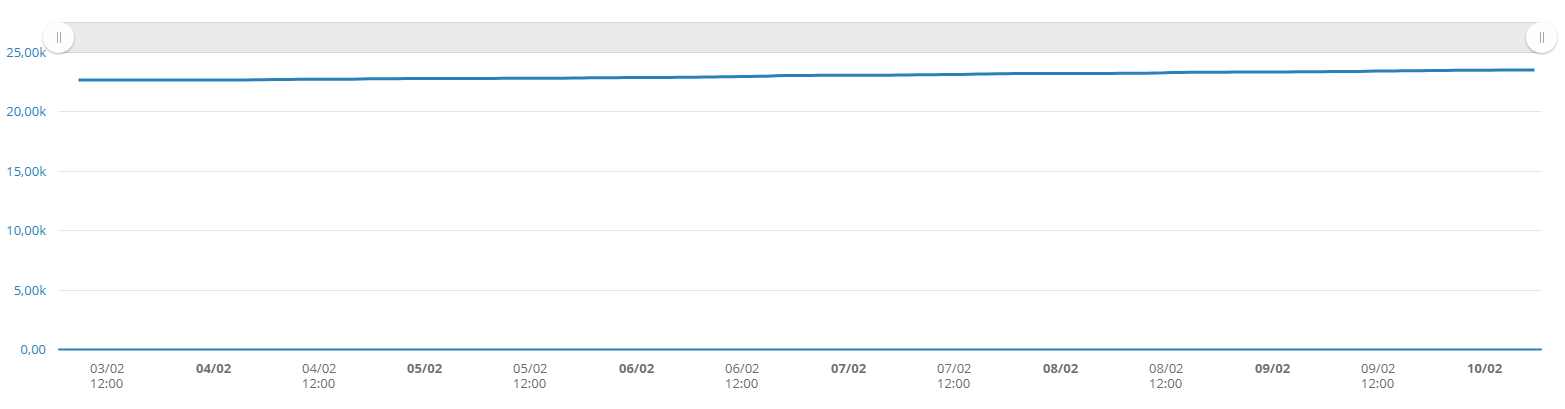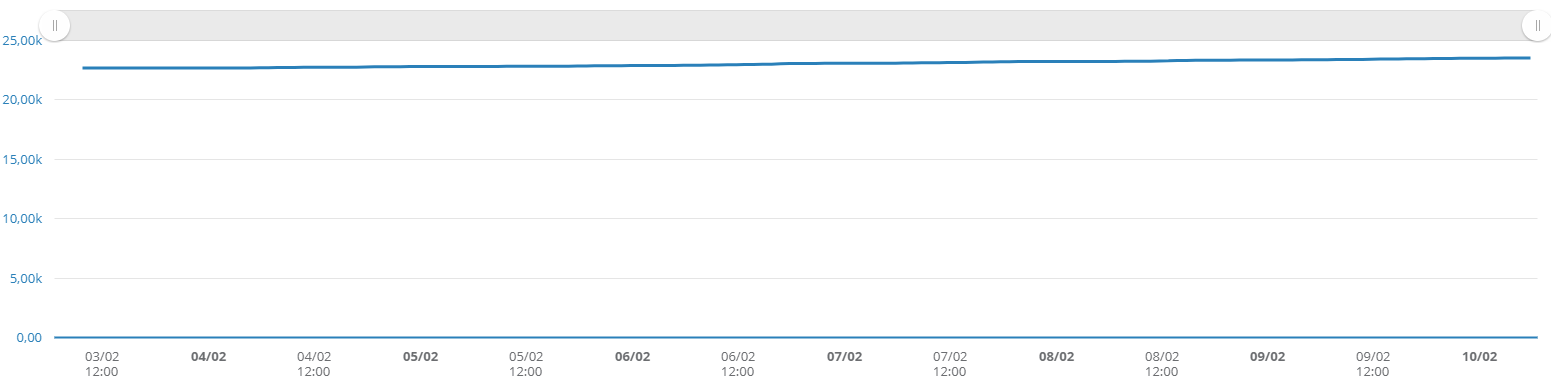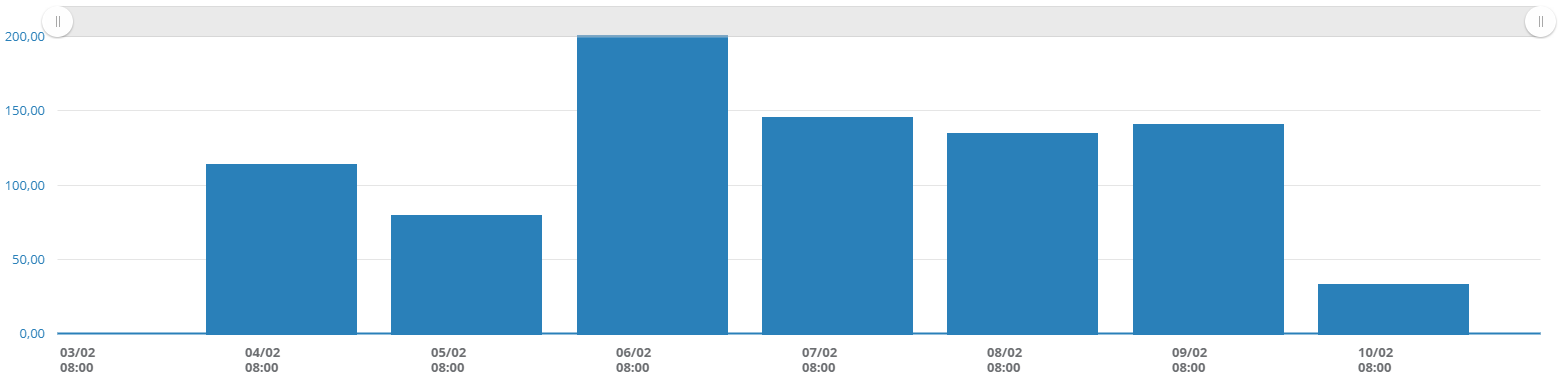# I have an incremental signal, how can I see the steps among values?

Scenario: the source signal is a simple signal (it's not a counter or a virtual signal) that represents an incremental value, at each transmission the total value of a counter is sent. The signal is not set as counter so the steps among values are not directly available.As first step to calculate a signal that represents the steps among values you need to

• create a virtual signal that represents the original signal shifted of one unit, to refer the previous valueThe virtual signal has to be created with the following formula

• kf(ago(x0),2)

Is therefore possible to

• create a virtual signal that calculate the difference between the source signal and the shifted one

By importing the two signals as variables in the virtual signal we'll have the original signal as x0 and the shifted signal as y0, the formula to calculate steps is

• x0 - y0

To conclude

• set the signal as differential counter since the received values represent the increases and not the total accumulatedDifferent frequencies (Hourly total, weekly total, ...) will be available only when the signal is cached (if the signal is not cached, a message is shown in its edit page in the virtual signal box).